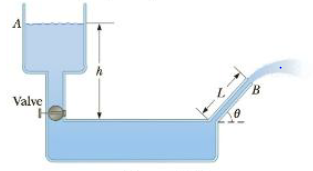Chapter 9, Problem 85AP

Chapter
Section
Textbook Problem

Figure P9.85 shows a water tank with a valve. If the valve is opened, what is the maximum height attained by the stream of water coming out of the right side of the tank? Assume h = 10.0 m, L = 2.00 m. and θ = 30.0°, and that the cross-sectional area at A is very large compared with that at B.Figure P9.85

To determine
The maximum height attained by the stream of water coming out of the right side of the tank.

Explanation

Given info: The height of the water level h from the valve is 10.0m , the length of the streamer is 2.00m , and the angle of the pipe L is 30.0° .

Explanation: Use Bernoulli’s equation to the points A and B by assuming the point B is at y=0 and the flow speed of water at point A is vA0 for the maximum height attained by the stream water. PA+0+ρwg(hLsinθ)=PB+(1/2ρwvB2+0) . The pressure at both points equal to the atmospheric pressure that is PA=PB=Patm . Solving these two expressions, the flow speed of water at point B is vB=2g(hLsinθ) .

To further solve the problem, incorporate the concept of projectile motion where v0y=vBsinθ and the velocity at point A is vy=0,y=ymax . Newton’s equations of motion is vy2=v0y2+2ay(Δy)ymax=(hLsinθ)sin2θ

Still sussing out bartleby?

Check out a sample textbook solution.

See a sample solution

The Solution to Your Study Problems

Bartleby provides explanations to thousands of textbook problems written by our experts, many with advanced degrees!

Get Started